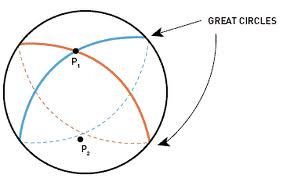Click to Chat

1800-1023-196

+91-120-4616500

CART 0

• 0

MY CART (5)

Use Coupon: CART20 and get 20% off on all online Study Material

ITEM
DETAILS
MRP
DISCOUNT
FINAL PRICE
Total Price: Rs.

There are no items in this cart.
Continue Shopping```SphereA sphere is a locus of a point which moves in space such that its distance from a fixed point is constant. Fixed point is called centre of sphere and constant distance is called radius of sphere. A sphere is the result of rotation of a circle about one of its parameters. Most of the properties and constituents of a sphere are similar to that of a circle.

There is a very minute difference between the properties of a sphere and a circle. We begin with some of the components of a sphere:

Some Important Terminology

A line segment joining two points on a sphere and passing through the centre is called a diameter.

The shortest possible distance between any two points on a sphere is termed as geodesic.

The surface area of the sphere of radius ‘r’ is given by A = 4πr2

The volume of the sphere of radius ‘r’ is given by V = 4/3 πr3

Great Circle:

?Great circle is an important concept associated with the sphere. The circle which is formed as a result of intersection of the surface of the sphere with a plane that passes through the center of the sphere. There can be infinite number of great circles satisfying a particular requirement.Equation of Sphere in Diverse Forms

Now we discuss the equation of sphere in various diverse forms:
The general equation of sphere with center at (a, b, c) and radius ‘r’ is given by

(x – a)2 + (y – b)2 + (z – c)2 = r2

The same general equation in expanded form can be written as

x2 + y2 + z2 + 2ux + 2vy + 2wz + d = 0

The center in this case becomes (–u, –v, –w),

And radius is given by r= √μ2 + v2 + w2 – d.

Remark:

If instead of (a, b, c), the centre is at origin i.e. (0, 0, 0) then the equation becomes x2 + y2 + z2 = r2.

In spherical coordinates, the points on the sphere can be written as

x = x0 + r cos θ sin φ

y = y0 + r cos θ sin φ

z = z0 + r cos φ where 0 ≤ θ ≤ 2π and 0 ≤ φ ≤ π

Diameter form:

By the diameter form we mean the equation of the sphere when extremities of the diameter are given.

Equation of a sphere whose extremities of diameter are A (x1, y1, z1) and B (x2, y2, z2) is

(x – x1) (x – x2) + (y – y1) (y – y2) + (z – z1) (z – z2) = 0.

You may also view the following video for more on sphere

We now illustrate some of the examples based on these concepts:

Illustration:

Find the equation of the sphere which passes through the points (1, –3, 4), (1, –5, 2) and (1, –3, 0) and whose centre is on the plane x + y + z = 0.

Solution:

Let equation of the sphere be

x2 + y2 + z2 + 2ux + 2vy + 2wz + d = 0

Its centre is (- u, - v, - w) which is on x + y + z = 0

⇒ u + v + w = 0

It passes through (1, - 3, 4) ⇒ 2u - 6v + 8w + d = - 26       … (2)

(1, - 5, 2) ⇒ 2 u - 10 v + 4 w + d = - 30   … (3)

and it passes through (1, - 3, 0) ⇒2u - 6v + d = - 10  … (4)

Solving these four equations we get,

u = - 1, v = 3, w = - 2 and d = 10

Therefore required equation of the sphere is

x2 + y2 + z2 - 2x + 6y - 4z + 10 = 0.

Illustration:

Find the equation of the sphere whose centre is (2, –3, 4) and which passes through the point (1, 2, –1).

Solution:

We know that the radius of the sphere is

Radius of sphere = √{(2–1)2 + (–3–2)2 + (4+1)2} = √51

∴ Equation of the sphere is (x – 2)2 + (y + 3)2 + (z – 4)2 = (√51)2

i.e. x2 + y2 + z2 – 4x + 6y – 8z – 22 = 0.

Students are advised to master these concepts in order to remain competitive in the IIT JEE and other engineering exams.

Related Resources

Look into the Past Year Papers with Solutions to get a hint of the kinds of questions asked in the exam.

You can get to know the Important Books of Mathematics here.

To read more, Buy study materials of 3D Geometry comprising study notes, revision notes, video lectures, previous year solved questions etc. Also browse for more study materials on Mathematics here.
```### Course Features

• 731 Video Lectures
• Revision Notes
• Previous Year Papers
• Mind Map
• Study Planner
• NCERT Solutions
• Discussion Forum
• Test paper with Video Solution